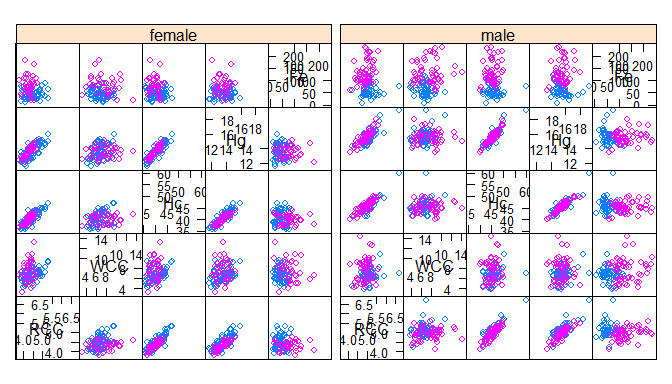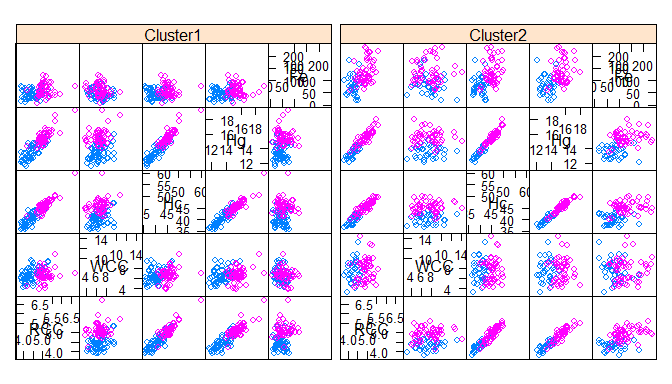# MoEClust: Gaussian Parsimonious Clustering Models with Gating and Expert Network Covariates

## Introduction

MoEClust is an R package which fits finite Gaussian Mixtures of Experts models using a range of parsimonious covariance parameterisations via the EM/CEM algorithm, ie. allows incorporation of covariates into the mixing proportions and/or Gaussian densities of finite Gaussian mixture models under the various parsimonious covariance parameterisations in the GPCM famly (e.g. mclust) These models were introduced by Murphy and Murphy (2017). The package also visualises Gaussian mixture of experts models with parsimonious covariance parameterisations using generalised pairs plots.

The most important function in the MoEClust package is: MoE_clust, for fitting the model via the EM/CEM algorithm with gating and/or expert network covariates, supplied via formula interfaces. Other functions also exist, e.g. MoE_control, MoE_crit, MoE_dens, MoE_estep, and aitken, which are all used within MoE_clust but are nonetheless made available for standalone use. MoE_compare is provided for conducting model selection between different results from MoE_clust using different covariate combinations &/or initialisation strategies, etc.

A dedicated plotting function exists for visualising the results using generalised pairs plots, for examining the gating network, and/or log-likelihood, and/or clustering uncertainties, and/or graphing model selection criteria values. The generalised pairs plots (MoE_gpairs) visualise all pairwise relationships between clustered response variables and associated continuous, categorical, and/or ordinal covariates in the gating &/or expert networks, coloured according to the MAP classification, and also give the marginal distributions of each variable (incl. the covariates) along the diagonal.

An as.Mclust method is provided to coerce the output of class "MoEClust" from MoE_clust to the "Mclust" class, to facilitate use of plotting and other functions for the "Mclust" class within the mclust package. As per mclust, MoEClust also facilitates modelling with an additional noise component (with or without the mixing proportion for the noise component depending on covariates). Finally, a predict method is provided for predicting the fitted response and probability of cluster membership (and by extension the MAP classification) for new data, in the form of new covariates and new response data, or new covariates only.

The package also contains two data sets: ais and CO2data.

If you find bugs or want to suggest new features please visit the MoEClust GitHub issues page. This vignette aims to demonstrate the MoEClust models via application to well-known univariate and multivariate data sets provided with the package.

### Installing MoEClust

MoEClust will run in Windows, Mac OS X or Linux. To install it you first need to install R. Installing Rstudio as a nice desktop environment for using R is also recommended.

Once in R you can type at the R command prompt:

install.packages('devtools')
devtools::install_github('Keefe-Murphy/MoEClust')

to install the latest development version of the package from the MoEClust GitHub page.

To instead install the latest stable official release of the package from CRAN go to R and type:

install.packages('MoEClust')

In either case, if you then type:

library(MoEClust)

it will load in all the MoEClust functions.

The GitHub version contains a few more features but some of these may not yet be fully tested, and occasionally this version might be liable to break when it is in the process of being updated.

## CO2 Data

data(CO2data)
GNP   <- CO2data[,1]
CO2   <- CO2data[,2]

Fit various MoEClust mixture models to cluster the CO2 data, allowing the GNP variable enter the gating &/or expert networks, or neither, via a formula interface. Note that for models with covariates in the gating network, or models with equal mixing proportions, we don’t need to fit single-component models (though it could be done!) as this would merely duplicate the single-component models within m1 and m3, respectively. For the model with no covariates, we can only fit a model with only a noise component by including G=0.

m1    <- MoE_clust(CO2, G=0:2, verbose=FALSE)
m2    <- MoE_clust(CO2, G=2,   gating= ~ GNP, verbose=FALSE)
m3    <- MoE_clust(CO2, G=1:2, expert= ~ GNP, verbose=FALSE)
m4    <- MoE_clust(CO2, G=2,   gating= ~ GNP, expert= ~ GNP, verbose=FALSE)
m5    <- MoE_clust(CO2, G=2,   equalPro=TRUE, verbose=FALSE)
m6    <- MoE_clust(CO2, G=2,   expert= ~ GNP, equalPro=TRUE, verbose=FALSE)

Choose the best model among these and examine the results.

(comp <- MoE_compare(m1, m2, m3, m4, m5, m6, pick=5))
## ------------------------------------------------------------------------------
## Comparison of Gaussian Parsimonious Clustering Models with Covariates
## Data: CO2
## ------------------------------------------------------------------------------
##
##  rank MoENames modelNames G df iters      bic      icl      aic  loglik gating expert equalPro
##     1       m3          V 2  7    11 -157.205 -160.036  -147.88  -66.94   None   ~GNP    FALSE
##     2       m3          E 2  6    13 -158.841 -162.497 -150.848 -69.424   None   ~GNP    FALSE
##     3       m4          V 2  8    19 -159.251 -161.465 -148.593 -66.297   ~GNP   ~GNP    FALSE
##     4       m4          E 2  7    13 -160.205 -163.765 -150.879  -68.44   ~GNP   ~GNP    FALSE
##     5       m6          E 2  5    10 -160.267 -164.488 -153.606 -71.803   None   ~GNP     TRUE

(best <- comp$optimal) ## Call: MoE_clust(data = CO2, G = 1:2, expert = ~GNP, verbose = FALSE) ## ## Best Model (according to BIC): univariate, unequal variance (V), with 2 components ## BIC = -157.205 | ICL = -160.036 | AIC = -147.88 ## Including expert network covariates: ## Expert: ~GNP (summ <- summary(best)) ## ------------------------------------------------------ ## Gaussian Parsimonious Clustering Model with Covariates ## Data: CO2 ## ------------------------------------------------------ ## ## MoEClust V (univariate, unequal variance), with 2 components ## ## Gating Network Covariates: None ## Expert Network Covariates: ~GNP ## Equal Mixing Proportions: FALSE ## Noise Component: FALSE ## ## log.likelihood n d df iters BIC ICL AIC ## -66.94 28 1 7 11 -157.205 -160.036 -147.88 ## ## Clustering table: ## 1 2 ## 6 22 Visualise the results for the optimal model using a generalised pairs plot. plot(comp$optimal, what="gpairs", jitter=FALSE)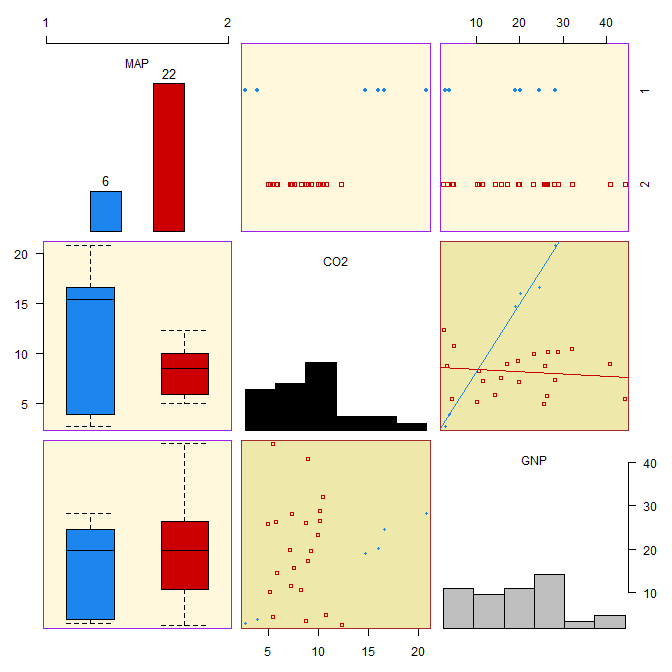Visualise the density of the mixture distribution.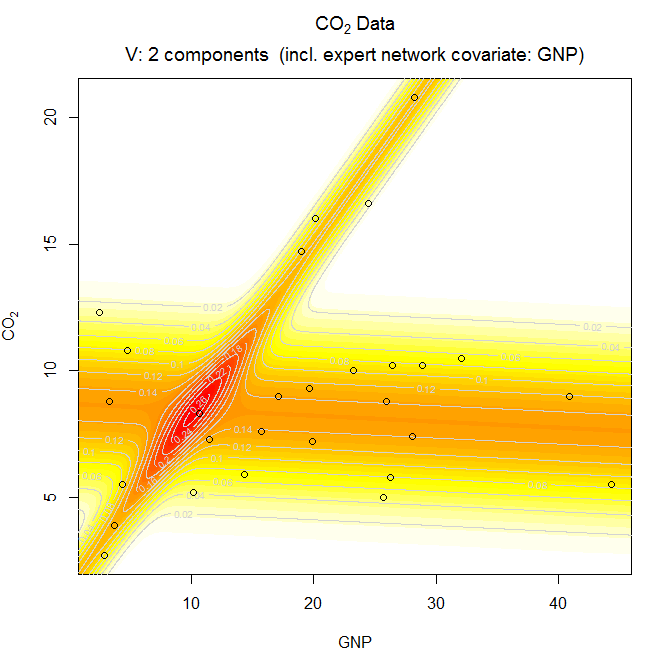Convert from the "MoEClust" class to the "Mclust" class in order to further visualise the results. Examine the "classification" and "uncertainty" options.

(mod <- as.Mclust(comp$optimal)) ## 'Mclust' model object: (V,2) ## ## Available components: ##  "call" "data" "modelName" "n" "d" "G" "BIC" "bic" "loglik" "df" "hypvol" "parameters" "z" "classification" "uncertainty" plot(mod, what="classification") plot(mod, what="uncertainty")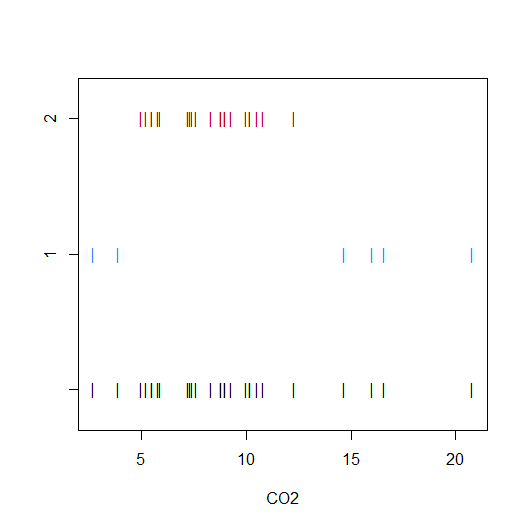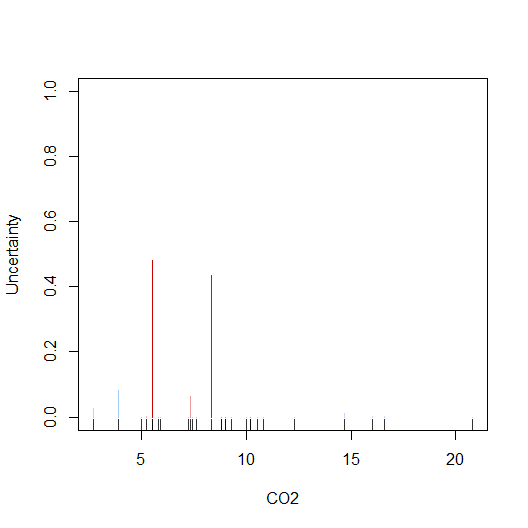Predictions can also be made from MoEClust models: the response, probability of cluster membership, and the MAP classification can be predicted for the fitted data or for new data (in the form of new covarariates and new response variables, or new covariates only). Let’s predict the response variable using the optimal model fit above to the CO2 data. as.vector(predict(comp$optimal)$y) ##  14.226428 4.279468 20.495267 7.723780 8.501542 14.995105 8.311552 8.022793 8.061713 8.567448 7.929803 8.136292 8.065675 8.005080 8.457902 6.545264 8.279177 8.214599 8.073366 17.988547 8.602639 8.440831 8.343480 8.078727 7.644308 3.461675 8.221125 8.621750 Now let’s build a model on some of the CO2 data and retain the indices of the withheld observations: ind <- sample(1:nrow(CO2data), 2) res2 <- MoE_clust(CO2data[-ind,]$CO2, G=2, expert=~GNP, network.data=CO2data[-ind,])

Now we can make predictions on the withheld data, either by using the withheld covariates only, or by also using the withheld response variables. Note that newdata can be either a list with component(s) new.x (and optionally new.y) or a single matrix/data.frame with the appropriate columns.

predict(res2, newdata= list(new.x=CO2data[ind,"GNP", drop=FALSE])) # Using new covariates only
## $y ## CO2 ## 1 11.181608 ## 2 6.564174 ## ##$classification
## 1 2
## 2 2
##
## $z ## Cluster1 Cluster2 ## [1,] 0.2743464 0.7256536 ## [2,] 0.2743464 0.7256536 predict(res2, newdata = CO2data[ind,]) # Using both new covariates & new response data ##$y
##        CO2
## 1 8.192813
## 2 8.006282
##
## $classification ## 1 2 ## 2 2 ## ##$z
##       Cluster1 Cluster2
##   3.021614e-14 1.000000
## 1 9.953047e-07 0.999999

## AIS Data

Load the Australian Institute of Sports data.

data(ais)
hema  <- ais[,3:7]

Examine the various additional options around initialisation of the algorithm:

?MoE_control

Accept the defaults, and fit a parsimonious Gaussian mixture of experts MoEClust model to the hematological variables within AIS data, supplying sex in the expert network and bmi in the gating network via formula interfaces. This time, allow the printing of messages to the screen.

mod   <- MoE_clust(hema, G=1:3, expert= ~ sex, gating= ~ BMI, network.data=ais)

Visualise the results for the optimal model using a generalised pairs plot.

plot(mod, what="gpairs")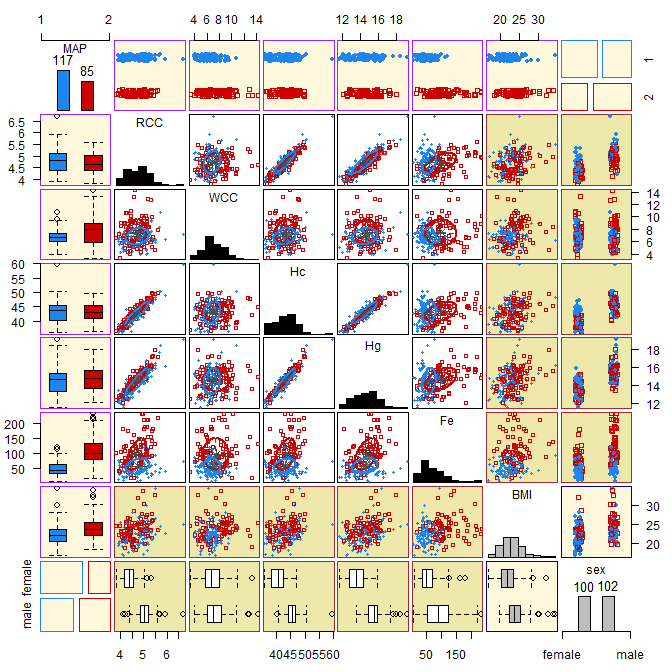Replace the scatter plots in response vs. response panels with bivariate density contours. Note that this is liable to be slow for models with expert network covariates.

plot(mod, what="gpairs", response.type="density")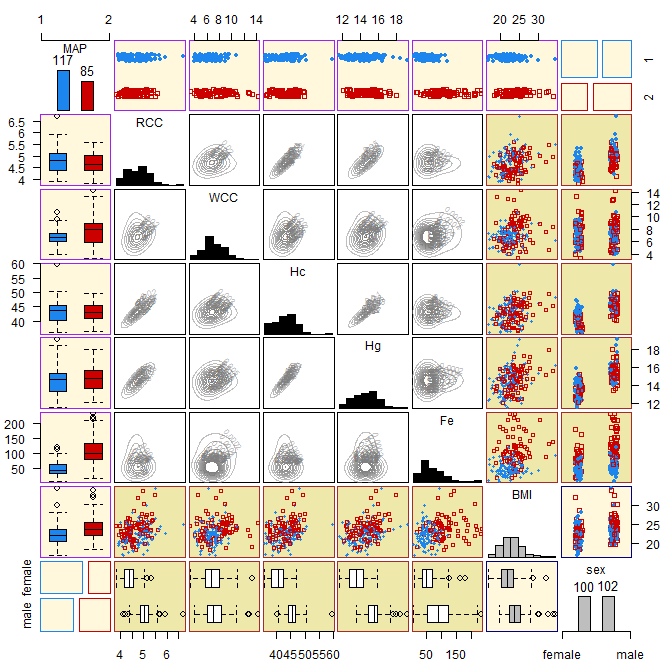Visualise the clustering uncertainty for the optimal model using a generalised pairs plot.

plot(mod, what="gpairs", response.type="uncertainty")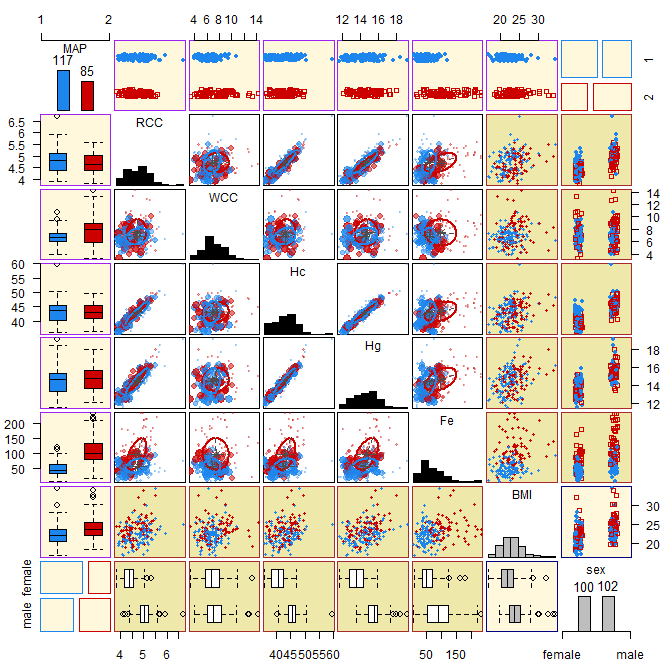Instead visualise the clustering uncertainty in the form of an ordered profile plot (type="barplot" can also be specified here).

plot(mod, what="uncertainty", type="profile")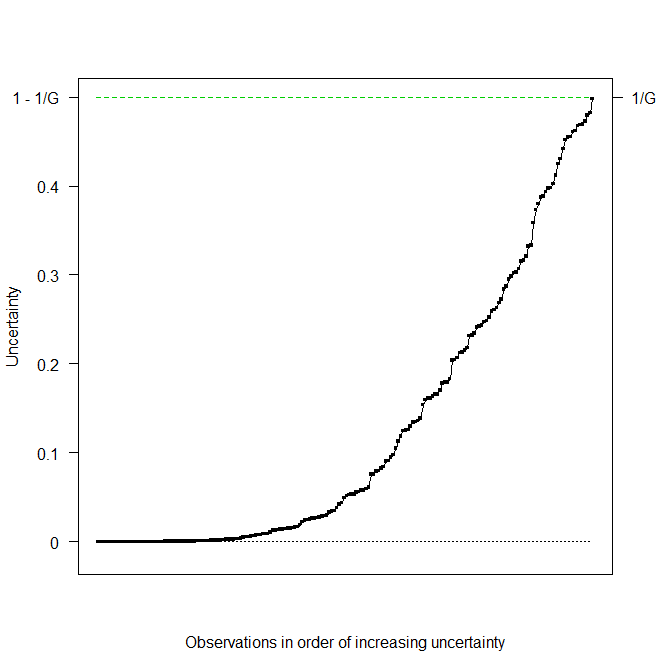Plot the BIC of the visited models.

plot(mod, what="criterion")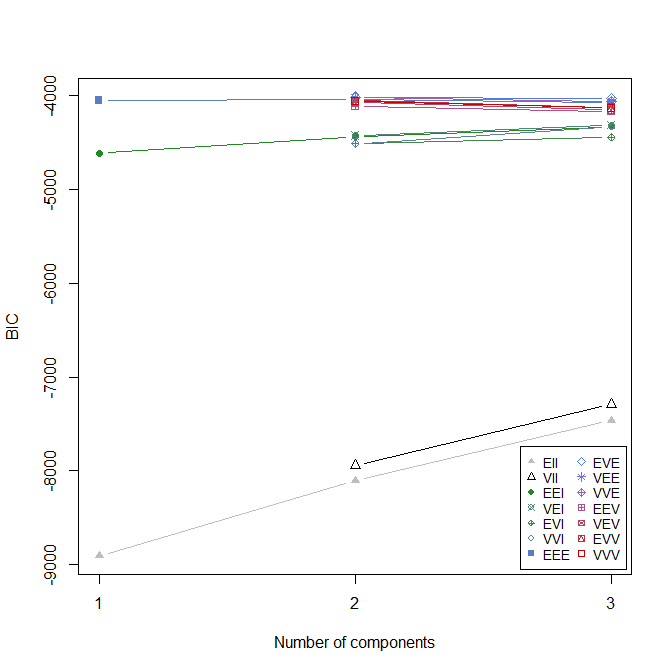For the optimal model, plot the gating network and the log-likelihood vs. EM iterations.

plot(mod, what="gating")
plot(mod, what="loglik")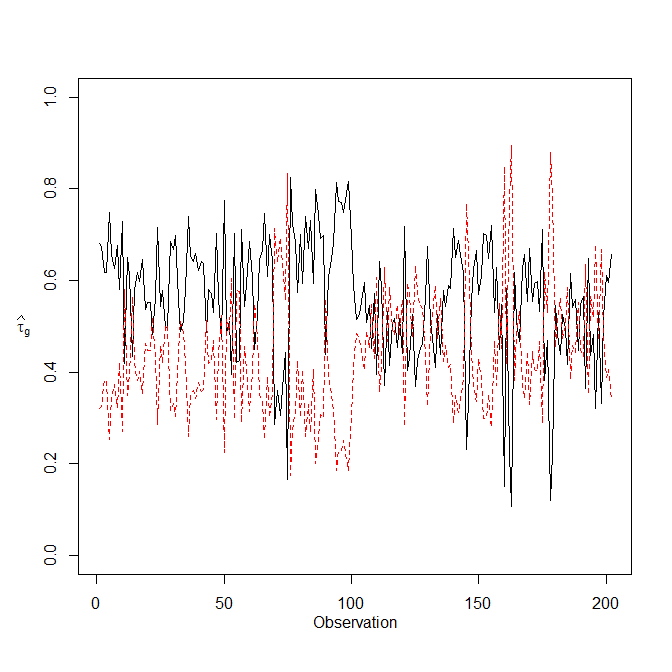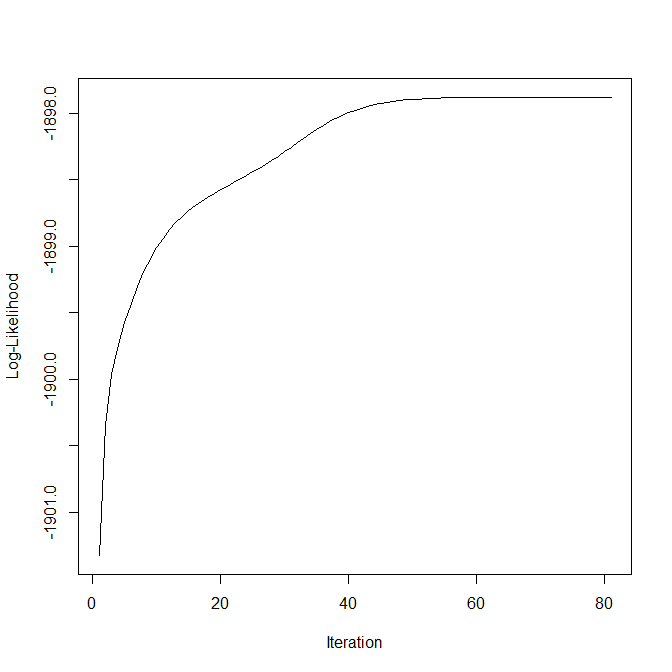Produce further visualisations with the aid of the lattice library.

require("lattice")
z <- factor(mod$classification, labels=paste0("Cluster", seq_len(mod$G)))
splom(~ hema | ais$sex, groups=z) splom(~ hema | z, groups=ais$sex)